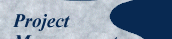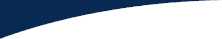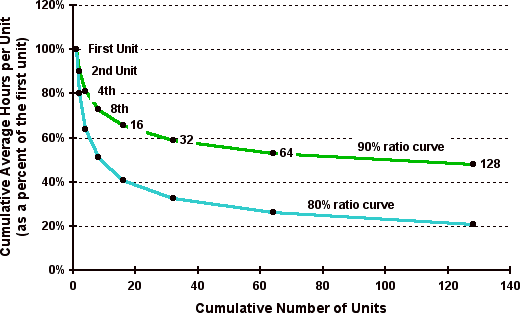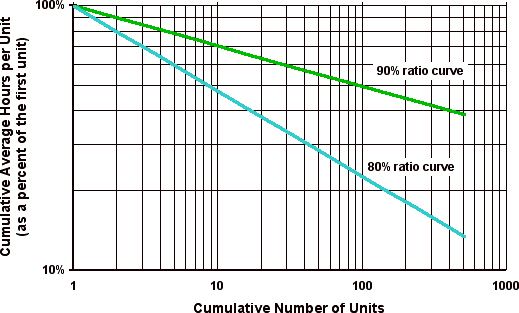This paper was first published in the Canadian Journal of Civil Engineering, Vol. 21, 1994 pp 939-953, under the title "A Pragmatic Approach to Using Resource Loading, Production and Learning Curves on Construction Projects". It has been modified only to the extent necessary to make it presentable in web page format. Published here October, 2001.

## Original Theory

The phenomenon of "learning" was first expressed mathematically in 1936 by T. P. Wright. He observed in the aircraft industry that certain costs per unit tend to decrease in a predictable pattern as the workers and their supervisors become more familiar with the work. These decreasing costs are a function of a learning process in which fewer and fewer man-hours are required to produce a unit of work as more and more units are produced. The key elements of the theory may be summarized as follows (Adrian 1987).

• The repetition of any task leads to an improvement in productivity as a result of the experienced gained.
• This phenomenon is well established in the mass production industry as well as in the construction industry under appropriate circumstances.
• The application of the theory (in the construction industry) assumes that operatives start with the necessary basic skills as well as the required support for the work to be accomplished.
• Productivity improvement then typically follows a constant ratio relationship which is expressed as follows.
For every doubling of units, the cumulative average time per unit is reduced by a constant ratio.

This relationship is illustrated in Table 1, showing examples of Cumulative Average Time per Unit (Chellew 1974).

 Number of Units in Sequence Cumulative Average Time per Unit 90% Ratio 80% Ratio 1 100.0 100.0 2 90.0 80.0 4 81.0 64.0 8 72.9 51.2 16 65.6 40.9 32 59.1 32.8
##### Table 1: Examples of Cumulative Average Time per Unit for Two Different Ratios

In Table 1, the time taken for the first unit is 100%. At a 90% ratio, the average time taken for the first and second unit is 90%, i.e., the actual time taken for the second unit is 80%. By the time the fourth unit is reached the average time taken for all four units is 90%x90% = 81% and so on.

These values can be plotted as curves as shown in Figure 9. However, if the same data is plotted on log-log paper as shown in Figure 10, the result is a straight line which is more useful for manual analysis or mathematical illustration.##### Figure 9: Illustration of learning curves